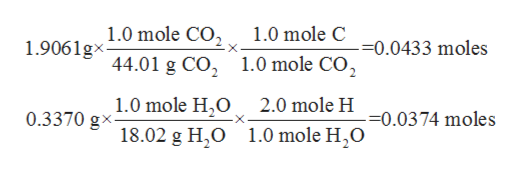# 1. A 0.7549g Sample of the compound burns in O2(g) to produce 1.9061g of CO2(g) and  0.3370 of H2O(g).a) calculate the individual masses of C, H, and O in the 0.7549 gram sample

Question
148 views

1. A 0.7549g Sample of the compound burns in O2(g) to produce 1.9061g of CO2(g) and  0.3370 of H2O(g).

a) calculate the individual masses of C, H, and O in the 0.7549 gram sample

check_circle

Step 1

The combustion reactions are a type of chemical reactions where hydrocarbons react with oxygen and result in the production of water and carbon dioxide.

Step 2

The number of moles of a given chemical species is defined as the ratio of mass to the molar mass of the substance. The mathematical formula is as shown below:

Step 3

The mass of the unknown compound is given to be 0.7549 g.

It burns to form 1.9061 g of carbon dioxide gas and 0.3370 g of water.

The molar mass of CO2 is 4...help_outlineImage Transcriptionclose1.0 mole CO2 1.0 mole C 1.9061g 44.01 g CO2 1.0 mole CO2 --0.0433 moles 1.0 mole H,O 2.0 mole H 0.3370 gx- -=0.0374 moles 1.0 mole H,O 18.02 g H,O fullscreen

### Want to see the full answer?

See Solution

#### Want to see this answer and more?

Solutions are written by subject experts who are available 24/7. Questions are typically answered within 1 hour.*

See Solution
*Response times may vary by subject and question.
Tagged in

### Physical Chemistry